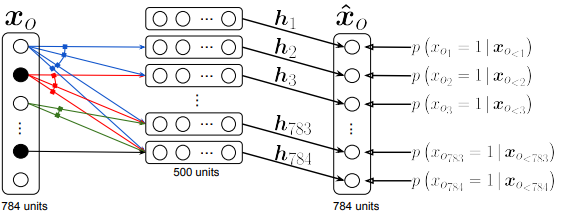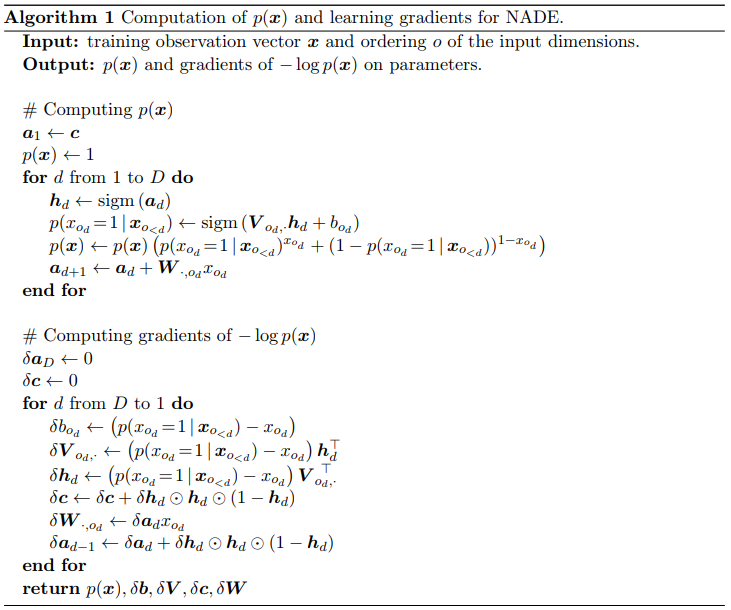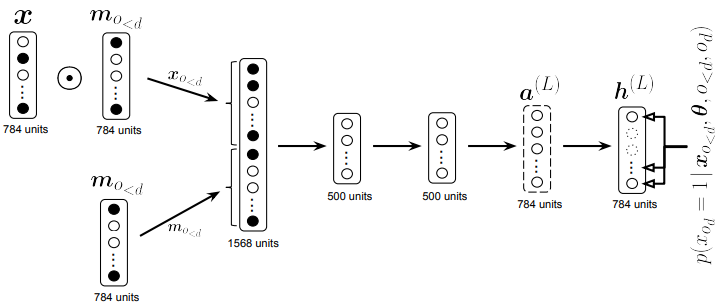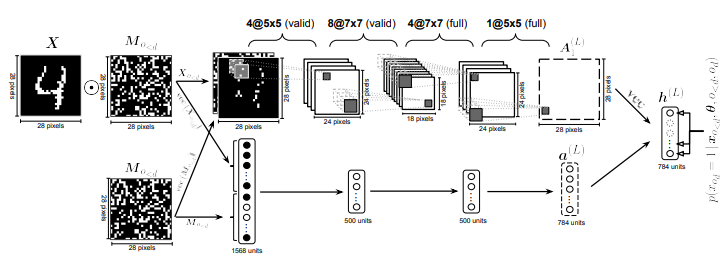# Neural Autoregressive Distribution Estimation

## WHY?

Estimating the distribution of data can help solving various predictive taskes. Approximately three approaches are available: Directed graphical models, undirected graphical models, and density estimation using autoregressive models and feed-forward neural network (NADE).

## WHAT?

Autoregressive generative model of data consists of D conditionals. NADE use feed-forward neural network to model each conditionals. First, we assume that x is binary. W and c are shared. p(x) = \prod_{d=1}^D p(x_{O_d})|x_{O_{<d}})\\ p(x_{o_d}|x_{o_{<d}}) = sigm(V_{o_d,\cdot}h_d + b_{o_d})\\ h_d = sigm(W_{\cdot, o<d}x_{o<d} + c)$% $ Computation cost is O(H) since W and c are shared. a_d = W_{\cdot, o_{<d}} + c\\ h_1 = sigm(a_1), where a_1 = c\\ h_d = sigm(a_d), where a_d = W_{\cdot, o_{<d}}x_{o_{<d}} + c = W_{\cdot, o_{d-1}}x_{o_{d-1}} + a_{d-1}$% $ NADE can be trained by maximum likelihood. Algorithm is as follows.We can extend NADE with other options. RNADE extends binary value x to real value, estimating rhe conditionals as Gaussian mixture.Orderless deep Nade mix the order of input using binary mask and concatenate mask as input to get conditionals.ConvNADE use convolution filters as W and stacks L layers.We can combine ConvNADE with deep NADE.

## So?

This paper performed extensive experiments with NADE. NADE was able to capture fairly good distribution of MNIST.

## Critic

Autoregressive framework is nice. However, I think this can be combined with hierarchical structure of features.

Powered by aiden